Home    |    Teacher    |    Parents    |    Glossary    |    About UsThe space that we live in has three dimensions: length, width, and height. Three-dimensional geometry, or space geometry, is used to describe the buildings we live and work in, the tools we work with, and the objects we create.

First, we'll look at some types of polyhedrons. A polyhedron is a three-dimensional figure that has polygons as its faces. Its name comes from the Greek "poly" meaning "many," and "hedra," meaning "faces." The ancient Greeks in the 4th century B.C. were brilliant geometers. They made important discoveries and consequently they got to name the objects they discovered. That's why geometric figures usually have Greek names!

We can relate some polyhedrons--and other space figures as well--to the two-dimensional figures that we're already familiar with. For example, if you move a vertical rectangle horizontally through space, you will create a rectangular or square prism.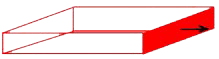If you move a vertical triangle horizontally, you generate a triangular prism. When made out of glass, this type of prism splits sunlight into the colors of the rainbow.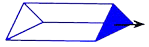Now let's look at some space figures that are not polyhedrons, but that are also related to familiar two-dimensional figures. What can we make from a circle? If you move the center of a circle on a straight line perpendicular to the circle, you will generate a cylinder. You know this shape--cylinders are used as pipes, columns, cans, musical instruments, and in many other applications.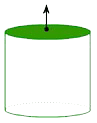A cone can be generated by twirling a right triangle around one of its legs. This is another familiar space figure with many applications in the real world. If you like ice cream, you're no doubt familiar with at least one of them!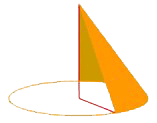A sphere is created when you twirl a circle around one of its diameters. This is one of our most common and familiar shapes--in fact, the very planet we live on is an almost perfect sphere! All of the points of a sphere are at the same distance from its center.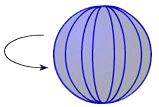There are many other space figures--an endless number, in fact. Some have names and some don't. Have you ever heard of a "rhombicosidodecahedron"? Some claim it's one of the most attractive of the 3-D figures, having equilateral triangles, squares, and regular pentagons for its surfaces. Geometry is a world unto itself, and we're just touching the surface of that world. In this unit, we'll stick with the most common space figures.

 Homework Help | Geometry | Three-dimensional figuresEmail this page to a friendSearch·  Space figures·  Prisms·  Pyramids·  Cylinders, cones     and spheresFirst Glance In Depth Examples WorkoutSpace figures